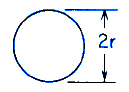Related Resources: calculators

### Torsional Deformation Circular Section Torsional Deformation Stress Equation and Calculator

Beam Deflection and Stress Equation and Calculators

Torsional Deformation and Stress Solid Circular Section Equations and CalculatorALL calculators require a Premium Membership

θ = TL / KG

K = 0.5 π r4

τmax = 2 T / π r3 at Boundary

Where:

θ - angle of twist (radians)
T = Twisting or torque moment force-length, (in-lbs, N-mm)
L = Length (in, mm)
τ = Unit shear stress force / area (lbs/in2, N/mm2)
G = Modulus of rigidity force / area (lbs/in2, N/mm2)
K = Polar Moment of Inertia (in4, mm4) for circular section
Q = Length cubed (in3, mm3)

Reference:
Roarks Formulas for Stress and Strain, 7th Edition, Table 10.1 Formulas for torsional deformation and stress.